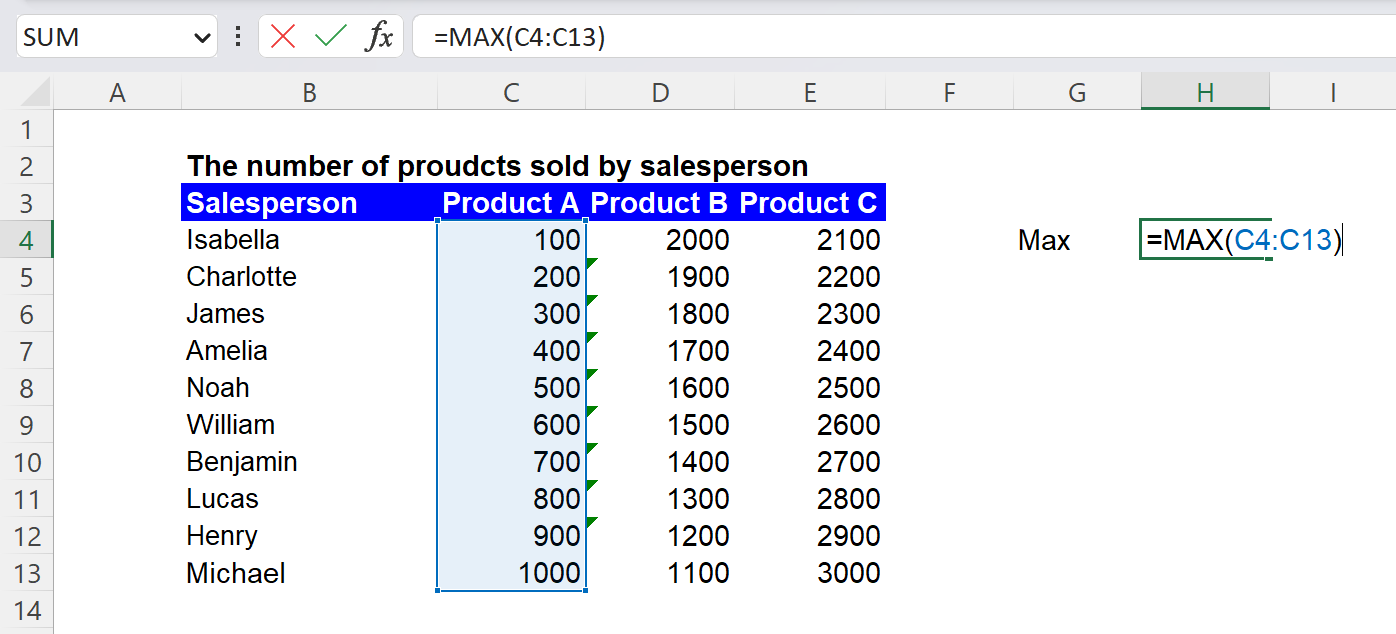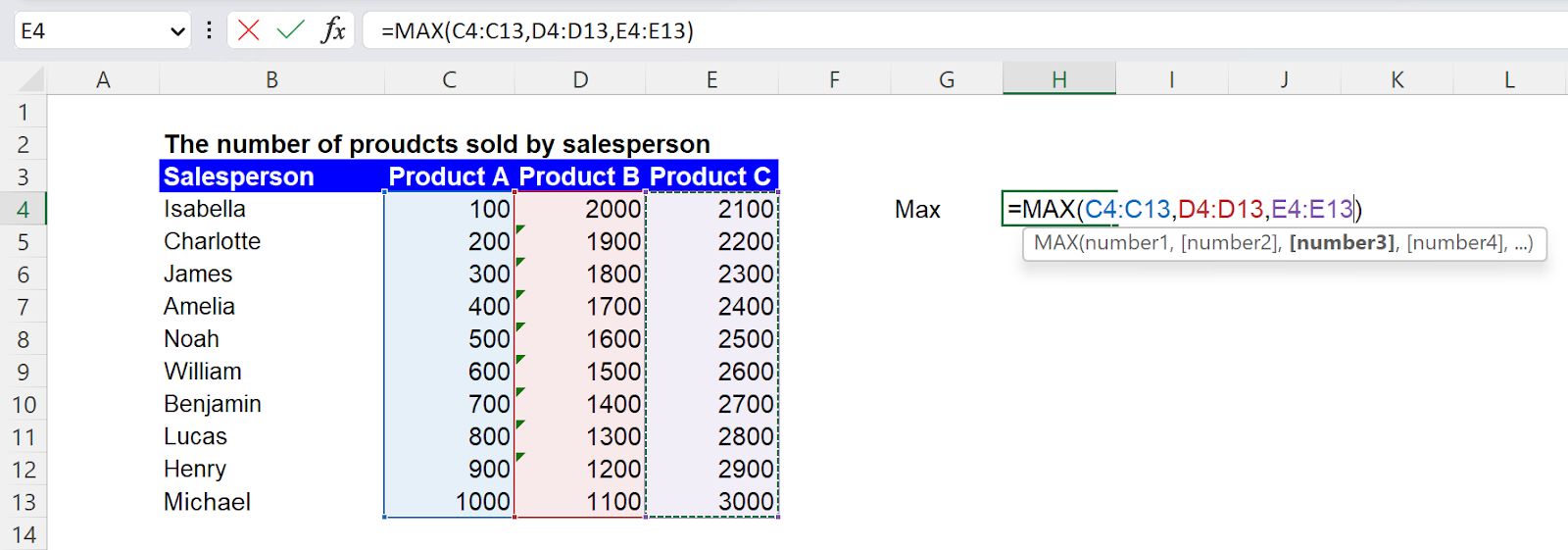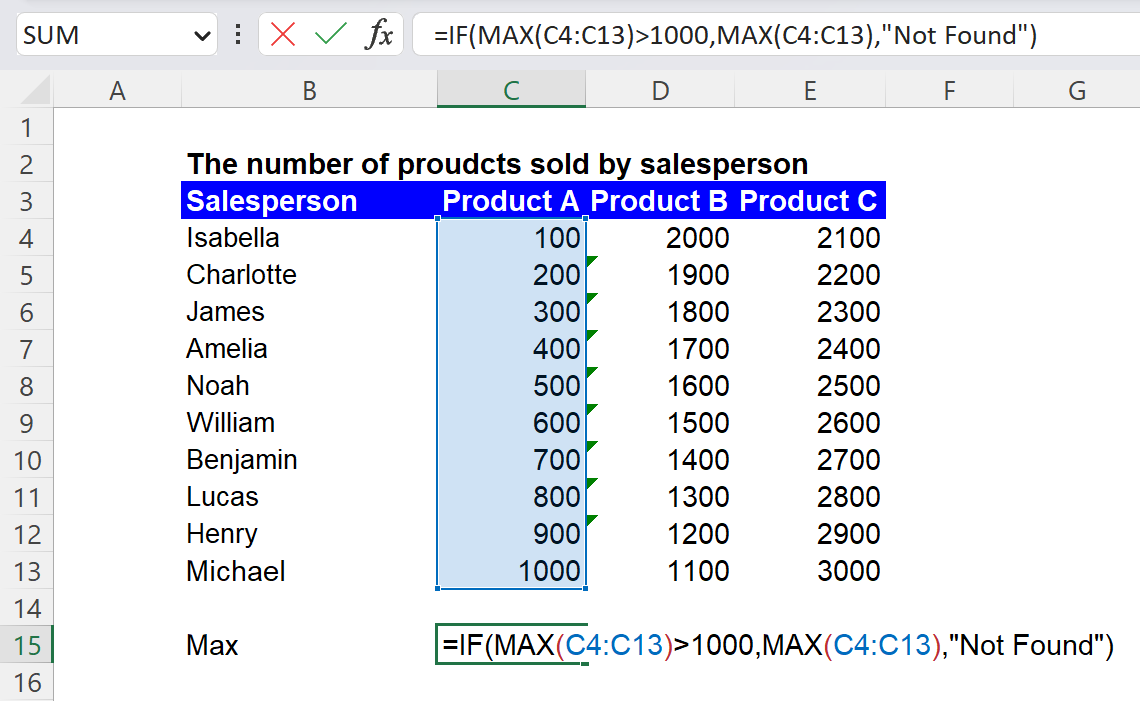Excel Formulas

# MAX Function in Excel: Explained

In this article, you will learn how to use the MAX Function in Excel.

## What is the MAX formula in Excel?

The MAX function in Excel is a built-in function that returns the most significant value from a range of cells or numbers.

## When is the MAX function beneficial in Excel?

The MAX formula can be helpful in various situations when working with data in Excel. Examples include:

1. Finding the highest value in a dataset: You can use the MAX formula to quickly identify the highest value in a column or row of data. This function can help determine the top salesperson in a team or the highest score on a test.
2. Comparing values: The MAX formula can be used in conjunction with other functions to compare values and make decisions. For instance, you could use the MAX formula to identify the highest value in a range of cells and then use the IF formula to check if that value meets a particular condition.

## How do you use the MAX function in Excel?

The MAX function in Excel is used by entering the function and its arguments into a cell. The syntax for the MAX function is:

``=MAX(number1, [number2], ...)``

number1: the first value or cell reference in which you want to find the maximum value

number2: you can select a cell, range, or an array as you do for “number1”

The formula returns the highest value among all input ranges.

Note: Empty cells, logical values, such as “TRUE” or “FALSE”, or text in the selected range are ignored by the MAX function. If the arguments don’t contain any numbers, the MAX formula returns zero.

For example, if you have a range of values in cells C4:C13 and want to find the maximum value in that range, you would enter the formula "=MAX(C4:C13)" in an empty cell. The formula returns 1000, the greatest value among the ten numbers.How to use the MAX formula with a range argument in Excel

You can also use the MAX function to find the maximum value of multiple ranges of cells by including multiple ranges as arguments, separated by commas, like this:

``=MAX(C4:C13, D4:D13, E4:E13)``

This formula returns 3000, which is the highest value in the selected field.How to use the MAX formula with multiple ranges argument in Excel

Or, you can select the entire array as follows:

``=MAX(C4:E13)``

You can also use the MAX function with other functions, such as IF and INDEX, to return a value based on a specific condition or to retrieve a value at a particular position in a range. For instance, you can use the MAX function together with an IF function to return the maximum value in a range only if it meets a certain condition, like this:

``=IF(MAX(C4:C13)>1000, MAX(C4:C13), "Not found")``

The value returned by the formula should be “Not found”, as no number is greater than 1000 in the selected range.How to use the MAX function with the IF formula in Excel

## What is the difference between the MAX and MAXIFS functions in Excel?

The MAX function and the MAXIFS formula in Excel are used to find the maximum value in a range of cells, but there is a key difference between the two. The MAX function returns the largest value from a range of cells or numbers. It takes one or more arguments, which can be a range of cells, multiple ranges of cells, or individual numbers. On the other hand, the MAXIFS formula allows you to find the maximum value in a range of cells based on one or more specified criteria.

## Analyze your live financial data in a snap in Google Sheets

Are you learning this formula to visualize financial data, build a financial model, or conduct financial analysis? In that case, LiveFlow may help you automate manual workflows, update numbers in real-time, and save time. You can access various financial templates on our website, from the simple Income Statement to Multi-Currency Consolidated Financial Statement. Are you interested in this product but are an Excel user? That’s not a problem at all. You can connect Google Sheets to Excel quickly.